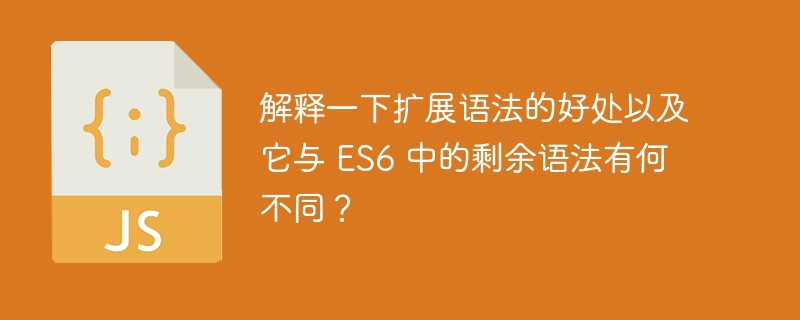# 解释一下扩展语法的好处以及它与 ES6 中的剩余语法有何不同？WBOY 转载
2023-09-19 21:21:03 397浏览### 传播语法

JavaScript 中的扩展语法是一种允许将可迭代对象（例如数组或对象）扩展为单个变量或元素的语法。

```let array = [10, 40, 7, 345];
let array2 = [...array];
```

### 扩展语法的好处

• 复制数组或对象，

• 合并数组或对象，以及

• 将多个元素作为函数参数传递。

### 示例

```<html>
<body>
<h2>Using the spread syntax to copy one array to another</h2>
<div id="output"></div>
<script>
let output = document.getElementById("output");
let array1 = [10, 40, 7, 345];
output.innerHTML += "Original array: " + array1 + "</br>";

// copy array using spread syntax
let array2 = [...array1];
output.innerHTML += "Copied array: " + array2 + "</br>";
</script>
</body>
</html>
```

### 示例

```<html>
<body>
<h2>Using the spread syntax to <i> copy one array to another</i></h2>
<div id="output"></div>
<script>
let output = document.getElementById("output");
let array = [10, 40, 7, 345];
output.innerHTML += "Array 1: " + array + "</br>";
let array2 = ["Hi, Hello"];
output.innerHTML += "Array 2: " + array2 + "</br>";
array = [...array2, ...array];
output.innerHTML += "After merging the array2 and array1: " + array + "<br/>";
</script>
</body>
</html>
```

### 示例

```<html>
<body>
<p> Passing multiple array elements as a function argument </p>
<div id="output"></div>
<script>
let output = document.getElementById("output");

// function to get a sum of 3 values
return param1 + param2 + param3;
}
let values = [50, 54, 72];

// passed array values using the spread syntax
output.innerHTML += "The sum of 3 array values: " + result + "</br>";
</script>
</body>
</html>
```

### 示例

```<html>
<body>
<h2>Using the spread syntax to <i>create a copy of the object.</i></h2>
<div id="output"></div>
<script>
let output = document.getElementById("output");
let sample_obj = {
name: "Shubham",
age: 22,
hobby: "writing",
};
let copy_object = {
...sample_obj,
};
output.innerHTML += "The values of the copy_object are " + copy_object.name + " , " +copy_object.age + " , " + copy_object.hobby +  "</br>";
</script>
</body>
</html>
```

## 剩余语法

### 语法

```function func(...params){

//params are the array of all arguments passed while calling the function

//users can access the params like params, params, params, ...
}
```

### 示例

```<html>
<body>
<h2>Using the rest syntax to collect function parameters</h2>
<div id="output"></div>
<script>
let output = document.getElementById("output");

// used the rest syntax to collect params
function mergeString(...params) {
let finalString = "";

// Iterating through the array of params
for (let param of params) {
finalString += param;
output.innerHTML += "Parameter: " + param + "<br>";
}
output.innerHTML += "The string after merging: " + finalString;
}
let strings = ["Welcome", "to", "the", "TutorialsPoint!"];

// used the spread syntax to pass all elements of // the strings array as an argument.
mergeString(...strings);
</script>
</body>
</html>
```

## 结论

JavaScript 中的扩展语法是一种允许将可迭代对象（例如数组或对象）扩展为单个变量或元素的语法。